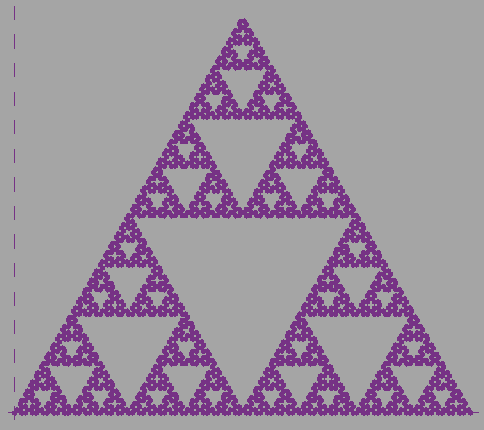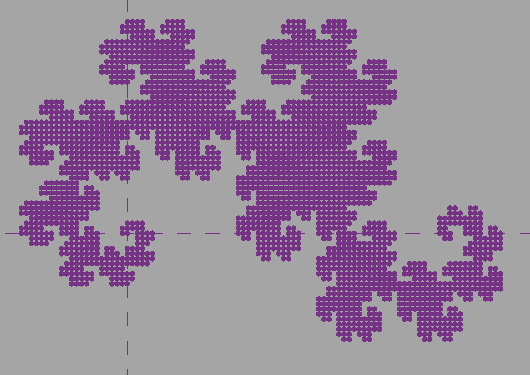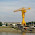## Thursday, August 22, 2013

### Dynamo Fractals

We've had a very productive and busy few days over here in the factory, but that hasn't stopped me from implementing something very dear to my heart; something I've been toying around with doing for a while now.

## What's a Fractal?

Fractals are geometric constructs consisting of self-similar patterns. Most, if not all fractals are generated using simple recursive rules. It is the recursive property of a fractal's definition that gives it it's unique self-similar structure. Wikipedia has a great article, as usual.

For my Dynamo exercise, I looked for fractals which lend themselves to a simpler geometric definition rather than a pixel-by-pixel definition (such as the Mandelbrot or Julia sets). The two I decided to implement are the Sierpiński Arrowhead and the Dragon Curve. Both of these fractals start as a simple geometric figure (a line) and then on each iteration of its recursive creation, all occurrences of the original geometric figure are replaced with a new figure that contains multiple occurrences of the original figure (which can then all be replaced in the next iteration).

These fractals can both be drawn by emulating a pen: we keep track of the direction the pen is facing, and we can make the pen draw lines of a certain length in its current direction. We can turn the pen, and we can draw with the pen. These can be emulated with Transformations: turning applies a rotation to the pen's transformation, and drawing adds a translation (and the end point of the draw operation can be stored as an XYZ for creating line geometry later).

One of the most famous fractals is the Sierpiński Triangle (also known as the Sierpiński Gasket). It's starting geometry is a solid equilateral triangle, and on each iteration an inverted equilateral triangle is removed from the center of the solid triangle, creating three solid equilateral triangles around the new hole.

The Sierpiński Arrowhead is used to approximate the whole triangle, and is useful for this exercise because it forms one complete curve (one can draw the entire Arrowhead without lifting up a pen). It's starting geometry is a line segment, that is replaced on each iteration with three line segments half the length of the original with 60 degrees between them. This replacement is inverted on every other application: the "bump" will alternate between being right-side-up and upside-down.

Based on this information, the algorithm looks like this:

Let:

• direction = direction of replacement
• length = length of each segment
• order = number of iterations to draw. Input by the user.
• scale = size of the total image. Input by the user.
In:
1. direction is initialized to upside-down. length is initialized to scale/(2^order). If order is odd, then turn 60 degrees (this will keep the triangle upright).
2. If order = 0: draw a line of length length.
3. Recur with order - 1 and direction flipped.
4. Turn 60 degrees in the current direction.
5. Recur with order - 1 and current direction.
6. Turn 60 degrees in the current direction.
7. Recur with order - 1 and direction flipped.
Step 1 is the initialization of a recursive loop, so in Dynamo all of this will be done in a node that wraps a recursive node.

The length initialization is calculated by dividing the starting scale by 2 for each iteration that will occur.

Step 2 is the start of a recursive function. All recursive calls will start there. Step 2 also represents the base case: if we started this algorithm with order as 0, then we would immediately draw a line of length scale along the X axis, which is the correct result.

Steps 3, 5, and 7 draw each of the three sub-sections of a single iteration, separated by turns in the current direction. This is exactly the definition of a single iteration of the arrowhead:  three sub-sections with 60 degrees between them. If order is 1, then the recursive calls will draw lines, and the result will be the one "bump", which is the correct result.

With that in mind, let's look at the Dynamo implementation.

At the top, we calculate the length of all drawn segments and create a translation transformation out of it. Rotation is initialized to -60 degrees. The starting transformation is set to either the identity transformation if the starting order is even, or a 60 degree rotation if the order is odd. We also initialize an accumulator that will store all of the results; since we will be storing the end-points of all the translations (emulating the draw function), we need to supply the starting point, which is the origin. All of this is passed to arrowhead curve, which contains the recursive loop.

Some notes on the inputs:
• order is the current order we are drawing
• result is the accumulated XYZs representing the end points of the drawn lines
• transform is the accumulated transformation that contains all of the translations and rotations corresponding to the draws and turns used to generate the XYZs
• translate is the pre-calculated translation used to draw the segments
• rotation is the rotation amount to be applied for turns in this iteration
If the order is 0, then we perform a draw by applying the translate to the current transform, and then applying this to an XYZ at the origin to get the appropriate XYZ. The XYZ is added to the accumulator, and the updated accumulator is returned. The updated transform with the translate applied is also returned.

If the order is not 0, the we draw the three sub-sections. We draw the first sub-section with the rotation flipped (which flips the direction). We take the result transform from the first sub-section and rotate it by the current rotation, which emulates the first turn. We pass this turned transform to the second sub-section, along with the current (non-flipped) rotation, and the results of the first sub-section. We turn the result transform again before drawing the third sub-section, which again uses the flipped rotation and the results from the second (and first) sub-section. For all three sub-sections, the order is subtracted by 1, which allows the recursion to approach the base case of order = 0. The results of the third sub-section drawing are then used as the results of the recursive branch.Sierpinski Arrowhead in Revit, order of 7.

## Dragon Curve

The Dragon Curve (also known as the Jurassic Park Curve) is another popular fractal. It is created using 45 and 90 degree angles: its starting geometry is a single line segment, and on each iteration, the single line segment is replaced by two smaller line segments of equal length joined at a 90 degree angle and oriented such that the first new segment forms a 45 degree angle with the original segment. Like the Sierpiński Arrowhead, these replacements alternate between right-side-up and upside-down.

The algorithm looks like this:

Let:
• direction = direction of replacement
• length = length of each segment
• order = number of iterations to draw. Input by the user.
• scale = size of the total image. Input by the user.
In:
1. direction is initialized to right-side-up. length is initialized to scale/2^(.5*order).
2. If order = 0: draw a line of length length.
3. Turn 45 degrees in the current direction.
4. Recur with order - 1 and direction = right-side-up.
5. Turn 90 degrees in the opposite direction.
6. Recur with order - 1 and direction = upside-down.
7. Turn 45 degrees in the current direction.
Step 1 is the initialization of a recursive loop, so in Dynamo all of this will be done in a node that wraps a recursive node.

The length initialization is calculated by dividing the starting scale by the square root of 2 for each iteration that will occur. (Square root of 2 is used because a  45-45-90 right-triangle with a hypotenuse of 1 yields a side-length of root-2 in accordance with the Pythagorean theorem).

Step 2 is the start of a recursive function. All recursive calls will start there. Step 2 also represents the base case: if we started this algorithm with order as 0, then we would immediately draw a line of length scale along the X axis, which is the correct result.

Steps 4 and 6 draw both sub-sections of a single iteration, separated by a 90 degrees turn in the opposite direction. Before and after the sub-sections are drawn, there is a 45 degrees turn in the current direction. This is exactly the definition of a single iteration of the arrowhead:  two sub-sections that form a  45-45-90 right-triangle with the original segment. The first sub-section is drawn right-side up, and the second is drawn upside-down. If order is 1, then the recursive calls will draw lines, and the result will be the one "bump", which is the correct result.

Now let's look at the Dynamo implementation.

This should look familiar if you've already seen the Sierpiński Arrowhead. At the top, we generate the translation transformation to be used for emulating draw. The transformation is initialized to the identity transform, the direction starts right-side-up, and the accumulator is initialized to contain the starting point.

Again, this should be familiar. The base case is set up identically: we perform a draw the same way as before. The recursive case is different but should be straight-forward after seeing the Arrowhead version: first we turn 45 degrees in in the current direction, then we draw the first sub-section right-side up, then we turn 90 degrees in the opposite direction, then we draw the second sub-section, and finally we turn 45 degrees in the current direction. Like last time, the accumulator and the transformation are passed along to the results.Dragon Curve in Revit, order of 12

1.2.I can't find the input node and output node in dynamo 0.6.2 ? Where are they?

1.Here:http://autodeskvasari.com/profiles/blogs/dynamo-turtle-graphics-and-lindenmayer-systems

3.Hi,
Are you going to update this for Dynamo 1.2?

1....as in Turtle Graphics?